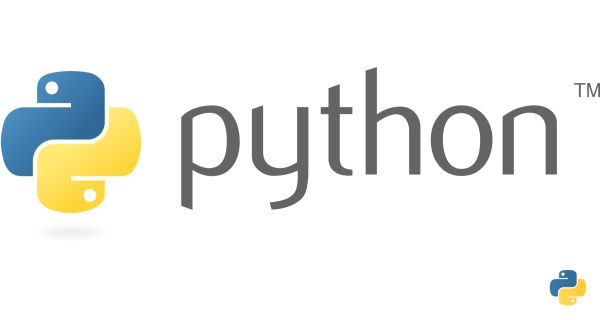# Recursive Function in Python## Understanding Recursive Functions

Recursive functions are functions that call themselves to solve a problem by breaking it down into smaller instances of the same problem. They are particularly useful when dealing with problems that exhibit repetitive structures or can be divided into smaller, similar components.

### How Recursive Functions Work

Let's understand the mechanics of recursive functions through a simple example:

Example:

``````def factorial(n):
if n == 0:
return 1
else:
return n * factorial(n - 1)``````

In this code snippet, the `factorial` function calculates the factorial of a non-negative integer `n`. The base case is when `n` is 0, in which case the function returns 1. Otherwise, it returns `n` multiplied by the factorial of `n - 1`.

### Example: Calculating Factorial Using Recursion

Let's calculate the factorial of 5 using the `factorial` function defined above:

``````result = factorial(5)
print("Factorial of 5:", result)``````

Output:

``Factorial of 5: 120``

Explanation:

Here's how the calculation takes place step by step:

1. `factorial(5)` calls `factorial(4)` and multiplies the result by 5.
2. `factorial(4)` calls `factorial(3)` and multiplies the result by 4.
3. This process continues until `factorial(0)` is reached, which returns 1.
4. The values are then propagated back up the call stack, resulting in the final result of 120.

• Elegance and Readability: Recursive solutions often closely mirror the problem's structure, leading to elegant and readable code.
• Complex Problem Solving: They are particularly well-suited for solving problems with inherent recursive properties, like traversing tree structures.
• Code Reusability: Recursive functions can be designed to solve similar sub-problems within the larger context of a problem.

### Common Mistakes and Considerations

When working with recursive functions, it's important to avoid common pitfalls:

• Base Case: Ensure the base case is well-defined to prevent infinite recursion.
• Optimization: Be cautious of redundant calculations. Memoization can be used to store and reuse already computed values.
• Memory Usage: Recursive calls consume memory. Deep recursion can lead to a stack overflow error.

### Conclusion

Recursive functions are a powerful tool in a programmer's toolkit, offering an elegant way to solve complex problems by breaking them down into smaller instances of the same problem. They enable us to write concise and intuitive code that mirrors the structure of the problem. However, it's crucial to use them judiciously, considering factors like base cases, optimization, and memory usage. With a solid understanding of recursive principles, you can leverage this technique effectively in your Python programming endeavors.